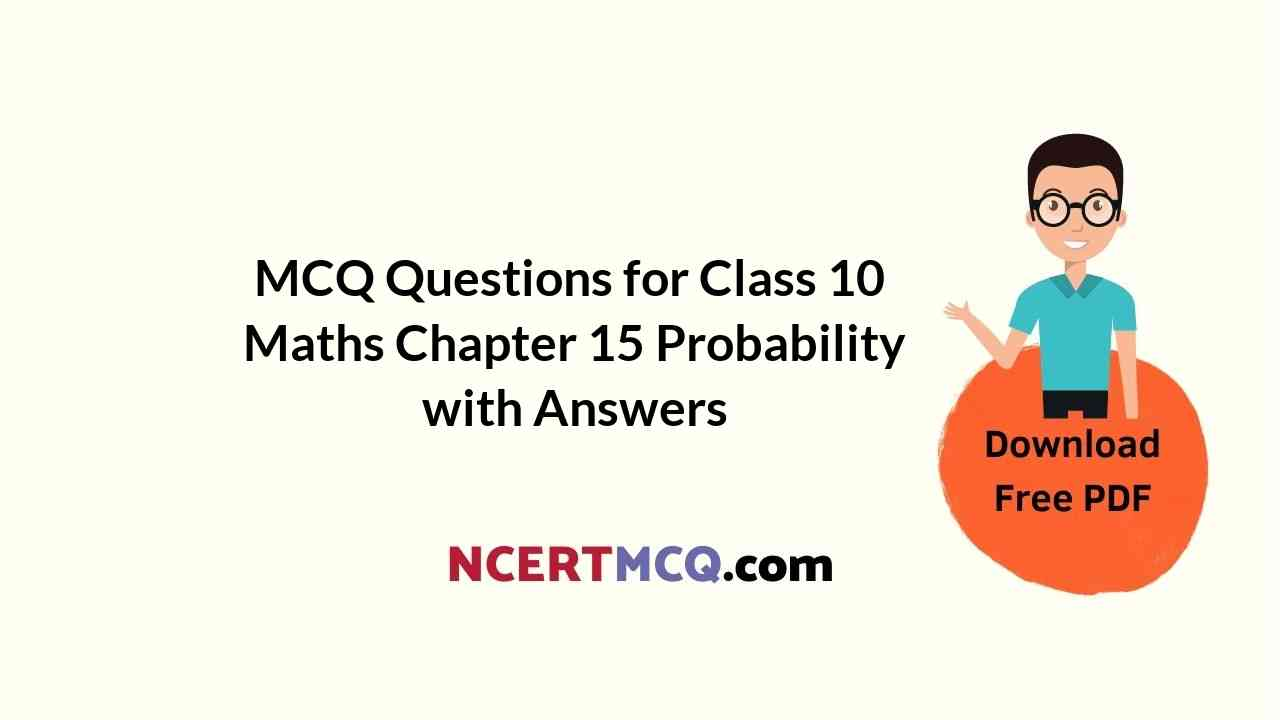Check the below NCERT MCQ Questions for Class 10 Maths Chapter 15 Probability with Answers Pdf free download. MCQ Questions for Class 10 Maths with Answers were prepared based on the latest exam pattern. We have provided Probability Class 10 Maths MCQs Questions with Answers to help students understand the concept very well. https://ncertmcq.com/mcq-questions-for-class-10-maths-with-answers/

Students can also refer to NCERT Solutions for Class 10 Maths Chapter 15 Probability for better exam preparation and score more marks.

## Probability Class 10 MCQs Questions with Answers

Probability Class 10 MCQ Question 1.
If two different dice are rolled together, the probability of getting an even number on both dice is:
(a) $$\frac{1}{36}$$
(b) $$\frac{1}{2}$$
(c) $$\frac{1}{6}$$
(d) $$\frac{1}{4}$$

Answer: (d) $$\frac{1}{4}$$

Probability MCQ Class 10 Question 2.
The probability that a number selected at random from the numbers 1, 2, 3, 4, …, 15 is a multiple of 4 is
(a) $$\frac{4}{15}$$
(b) $$\frac{2}{15}$$
(c) $$\frac{1}{5}$$
(d) $$\frac{1}{3}$$

Answer: (c) $$\frac{1}{5}$$

Class 10 Probability MCQ Question 3.
An event is very unlikely to happen. Its probability is closest to:
(a) 0.0001
(b) 0.001
(c) 0.01
(d) 0.1MCQ Of Probability Class 10 Question 4.
If the probability of an event is P, the probability of its complementary event will be:
(a) P – 1
(b) P
(c) 1 – p
(d) 1 – $$\frac{1}{p}$$

MCQ On Probability Class 10 Question 5.
In a family of 3 children, the probability of having atleast one boy is:
(a) $$\frac{7}{8}$$
(b) $$\frac{1}{8}$$
(c) $$\frac{5}{8}$$
(d) $$\frac{3}{4}$$

Answer: (d) $$\frac{3}{4}$$

Class 10 Maths Chapter 15 MCQ Question 6.
If P(A) denotes the probability of an event then:
(a) P(A) < 0
(b) P(A) > 0
(c) 0 ≤ P(A) ≤ 1
(d) -1 ≤ P(A) ≤ 0

Answer: (c) 0 ≤ P(A) ≤ 1

Ch 15 Maths Class 10 MCQ Question 7.
A card is selected from a deck of 52 cards. The probability of its being a red face card is:
(a) $$\frac{3}{26}$$
(b) $$\frac{3}{13}$$
(c) $$\frac{2}{13}$$
(d) $$\frac{1}{2}$$

Answer: (a) $$\frac{3}{26}$$

Probability Class 10 MCQ With Answers Question 8.
The probability than a non-leap year selected at random will contain 53 Sundays is:
(a) $$\frac{1}{7}$$
(b) $$\frac{2}{7}$$
(c) $$\frac{3}{7}$$
(d) $$\frac{5}{7}$$

Answer: (a) $$\frac{1}{7}$$

MCQ Probability Class 10 Question 9.
When a die is thrown, the probability of getting an odd number less them 3 is:
(a) $$\frac{1}{6}$$
(b) $$\frac{1}{3}$$
(c) $$\frac{1}{2}$$
(d) 0

Answer: (a) $$\frac{1}{6}$$

Probability Class 10 MCQs Question 10.
A card is drawn from a deck of 52 cards. The event E is that card is not an ace of hearts. The number of outcomes favourable to E is:
(a) 4
(b) 13
(c) 48
(d) 51

Class 10 Maths Ch 15 MCQ Question 11.
The probability of getting a bad egg in a lot of 400 is 0.035. The number of bad eggs in the lot is:
(a) 7
(b) 14
(c) 21
(d) 28

Chapter 15 Maths Class 10 MCQ Question 12.
A girl calculate that the probability of her winning the first prize in a lottery is 0.08. If 6000 tickets are sold, how many tickets has she bought?
(a) 40
(b) 240
(c) 480
(d) 750MCQs On Probability Class 10 Question 13.
One ticket is drawn at random from a bag containing tickets numbered 1 to 40. The probability that the selected ticket has a number which is a multiple of 5 is:
(a) $$\frac{1}{5}$$
(b) $$\frac{3}{5}$$
(c) $$\frac{4}{5}$$
(d) $$\frac{1}{3}$$

Answer: (a) $$\frac{1}{5}$$

Class 10 Maths Probability MCQs Question 14.
Someone is asked to take a number from 1 to 100. The probability that it is a prime is:
(a) $$\frac{1}{5}$$
(b) $$\frac{6}{25}$$
(c) $$\frac{1}{4}$$
(d) $$\frac{13}{50}$$

Answer: (c) $$\frac{1}{4}$$

Class 10 Maths Probability MCQ Question 15.
A school has five houses A, B, C, D and E. A class has 23 students, 4 from house A, 8 from house B, 5 from house C, 2 from house D and rest from house E. A single student is selected at random to be the class monitor. The probability that the selected student is not from A, B and C is:
(a) $$\frac{4}{23}$$
(b) $$\frac{6}{23}$$
(c) $$\frac{8}{23}$$
(d) $$\frac{17}{23}$$

Answer: (b) $$\frac{6}{23}$$